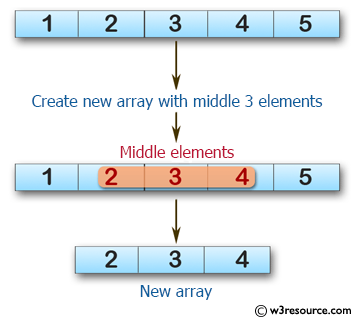﻿ Swift Array Programming Exercise: Create a new array of length 3 containing the elements from the middle of a given array of integers and length will be at least 3 - w3resource# Swift Array Programming Exercises: Create a new array of length 3 containing the elements from the middle of a given array of integers and length will be at least 3

## Swift Array Programming: Exercise-20 with Solution

Write a Swift program to create a new array of length 3 containing the elements from the middle of a given array of integers and length will be at least 3.

Pictorial Presentation:Sample Solution:

Swift Code:

`````` func three_elements(_ a: [Int]) -> [Int] {
var new_array = a

if a.count % 2 == 1 {
let ctr = a.count
let second_index = ctr / 2
let third_index = second_index + 1
let first_index = second_index - 1

new_array = [a[first_index], a[second_index], a[third_index]]
}

return new_array
}

print(three_elements([1, 2, 3, 4, 5]))
print(three_elements([7, 5, 4, 1, 3, 0, 9]))
print(three_elements([0, 1, 2]))
```
```

Sample Output:

```[2, 3, 4]
[4, 1, 3]
[0, 1, 2]
```

Swift Programming Code Editor:

Improve this sample solution and post your code through Disqus

What is the difficulty level of this exercise?

﻿